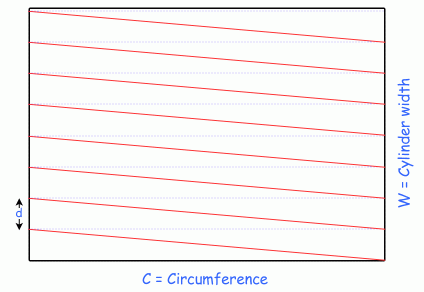SEARCH HOMEMath Central Quandaries & QueriesQuestion from john: I have a cylinder that is 37.5" width X 25.25" circumference. I need to create a repeating diagonal lined pattern on the cylinder so that when it prints it joins perfectly at the circumference repeat. The design must follow these specs. Black diagonal lines need to be 1.250" max width X 1.5" max spacing between the black lines. Please HelpHi John.

If we unroll the pattern of the cylinder, we have the following diagram:We won't worry about the thickness of the lines - that can be accomodated by simply centering the thickness around the pattern lines.

You can choose the value of d that is convenient for you (from your description that is up to 2.75" maximum). The actual width of the cylinder doesn't matter: you can start at one corner and then repeat the pattern.

Thus, you don't really need any more information than you already have: you can just mark off the distance d along the width of the cylinder and join the ends in straight lines.

If you want to know the angle formed by the red pattern line and the edge of the cylinder, you can use trigonometry's tangent function (tan).

Tan relates the size of an angle in a right triangle to the lengths of the sides opposite and adjacent to the angle. Thus,

tan θ = d/C so θ = arctan(d/C).

For example, if you use 2.5" for d and 25.25" for C, you get θ = 5.65 degrees.

Hope this helps,
Stephen La Rocque.Math Central is supported by the University of Regina and The Pacific Institute for the Mathematical Sciences.